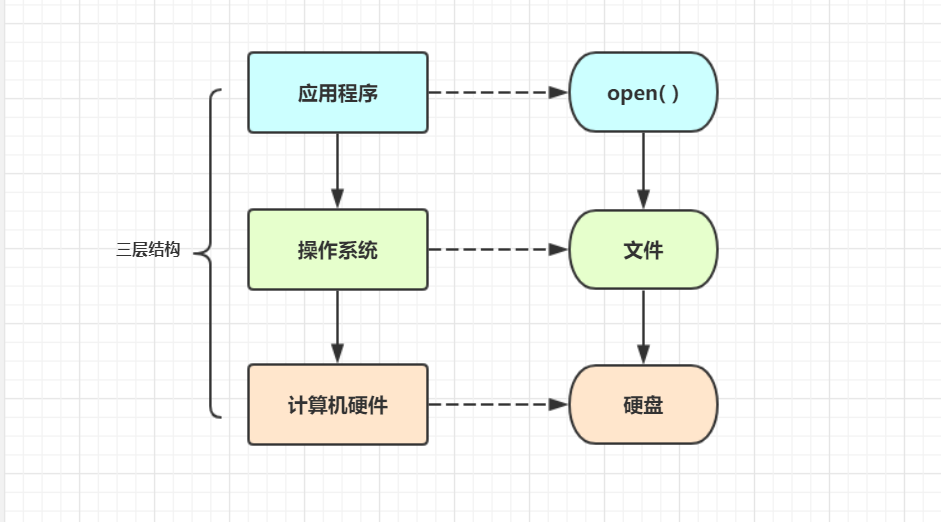# 19 文件处理

77次阅读

## 引入

### 1. 什么是文件

• 文件是操作系统提供给用户 / 应用程序存取硬盘的一种机制

• 永久保存数据

### 3. 如何使用文件

1. 应用程序调用 open() 功能, 拿到一个文件对象(文件句柄)
3. 然后操作系统将其转换成具体的 硬盘操作, 控制其怎么转## 一. 文件操作的步骤

### 1. 打开文件

• 使用 open() 向操作系统发起系统调用
• f : 文件对象(文件句柄), 相当于一个手柄, 用来远程操控文件的
• "r" : raw_str，指的是原生得字符串，里面得反斜杠 '\'，都会被视为普通字符
• 'a.txt' : 这是一个文件路径, 可以使用绝对路径(任何地方都可以找得到), 也可以使用相对路径 (在当前执行文件所在的目录下找)
• 'rt' : 文件打开的模式, "r" 是只读模式, "t" 是文本模式
• 'utf-8' : 字符编码
``````f = open(r'a.txt',mode='rt',encoding='utf-8')
``````

### 2. 操作文件

``````res = f = read()
print(res)
``````

### 3. 关闭文件 / 回收系统资源

• 通知操作系统 回收 刚刚读入的文件所占用的 内存空间
• 文件打开如果没有关闭, 操作系统会 过一段时间 清除 这个文件
• 对于我们来讲这么点时间不打紧, 但对于计算机来说就是占用它的资源 : 内存得不到释放
• 并且操作系统打开文件的数量是有限的, 打开的 文件数量过多 就会 影响电脑性能
``````f.close()
del f  #解除变量名绑定关系
``````

ps : 当文件关闭之后, 那么对应的文件操作的方法都不起作用了, 如果继续使用操作文件的功能就会 报错

ps2 : 当 f.close() 之后, 这个 "f" 文件对象并没有回收, 回收的是操作系统打开的文件资源

`````` f = open 占用了 2 部分的内存空间：🍉open 占用的是操作系统的的内存空间, 由操作系统的映射到了应用程序的内存空间中 ".close()关闭的就是这个 "
🍉"f" 的值还是一种变量，而变量都是归属于 python 的内存空间，也就是说 "f" 占用的是应用程序的内存空间
``````

## 二.with 上下文管理

### 1. 什么是 with

• with称之为：上下文管理，上文帮你打开文件，下文帮你关闭文件

### 2.with 打开单个文件

``````with open('a.txt', "rt", encoding="utf-8") as f1:
print(res)
# 哈哈哈
# 哈哈哈
🍉没有指定 "f.close()", "with" 会等其子代码块群不运行后，会自动调用 "f.close()"
``````

### 3.with 打开多个文件

``````🍉当你的一行代码过长，可以使用右斜杠 " \ " 转意换行符 "\n"(相当于是一行)
with open('a.txt', "at", encoding="utf-8") as f1, \
open("a.txt", "rt", encoding="utf-8") as f2:
res = f1.write(" 呵呵呵 \n")
print(res)
# 哈哈哈
# 哈哈哈
# 呵呵呵
``````

## 三. 文件的打开模式

• r : 只读 (默认)
• w : 只写
• a : 只追加写

### 3.encoding 编码格式与`t`, `b` 的关系

• 如果是 `t` 模式，必须加上 encoding="[编码格式]"
• 如果是 `b` 模式，不能加上 encoding="[编码格式]"

### 4. 示例

• #### `r` : 只读 (默认)

``````🍉'r'：在文件存在的时候，文件指针调到文件开头，文件不存在直接报错
f=open("a.txt",mode="rt",encoding='utf-8')
print(res)
f.close()
``````
• #### `w` : 只写

``````🍉'w'：在文件存在的时候会清空文件，文件指针调到文件开头，文件不存在会创建空文档
f=open("c.txt",mode="wt",encoding='utf-8')
# f.write("cccc\n")
f.write("11111\n")
f.close()
``````
• #### `a` : 只追加写

``````🍉'a'：在文件存在的时候不会清空文件，文件指针调到文件末尾，文件不存在会创建空文档
f=open("d.txt",mode="at",encoding='utf-8')
f.write("6666666\n")
f.write("11111\n")
f.close()
``````

### 5.`a` 模式与 `w` 模式区别

• 相同之处：在文件打开不关闭的情况下，连续的写入，新写的内容总会跟在之前写的内容之后
• 不同之处：
• `a` 模式重新开打文件，只要打开文件，它的指针就会跳到末尾 (通常用来记录日志文件)
• `w` 模式重新打开文件，直接清空文件 (通常用来创建新文件)

### 6. 可读可写: `+` 模式

• `r+` : 默认 t 模式, 读模式并加了它所没有的功能 (就是 "" 功能)
• `w+t` : 写模式, 加了它所没有的功能 (就是 "" 功能)
• `a+t` : 追加写, 加了它所没有的功能 (就是 "" 功能)
• `r+b` : b 模式, 读 + 写
• `w+b` : b 模式, 写 + 读
• `a+b` : b 模式, 追加写 + 读

## 四. 文件操作的其他方法

• .readlines() : 全部读出来, 存成列表的形式 (以换行符区分一行)
``````🍉".readline( )"
f=open('a.txt',mode='r',encoding='utf-8')
f.close()

f=open('a.txt',mode='r',encoding='utf-8') #mode='rt'
f.close()
``````

### 2、.writelines()

``````🍉循环从列表里面取出值写进去
f.writelines(['111111\n','222222\n','333333\n'])
f.write('aaaaaaaa\nbbbbbbb\nccccccc\n')  #.write() 加转行符号效果相同

f.close()
``````

### 2、.flush()

• 执行这个功能, 将会告诉操作系统 : 把内存缓冲区的数据立即刷入硬盘中
• 针对的是写操作, 主要用于测试行为
``````with open('aaa.txt', mode='wt', encoding='utf-8') as f:
f.write('I am Shawn')
f.flush()  # 立刻将文件内容从内存刷入硬盘
``````

### 3. 其他操作

• .writeable() : 判断是否可写
• .encoding : 查看文件对象使用的字符编码格式 ("b" 模式没有该功能)
• .name : 查看文件对象所对应的文件名
• .colsed : 判断文件是否关闭
``````with open('aaa.txt', mode='wt', encoding='utf-8') as f:
print(f.writable())  # True
print(f.encoding)    # utf-8
print(f.name)        # aaa.txt
print(f.closed)      # False
``````

ps : 如果没有指定 encoding，默认使用操作系统的编码，在 windsow 中显示：CP936 (其实就是 GBK）

## 六. 文件的修改方式